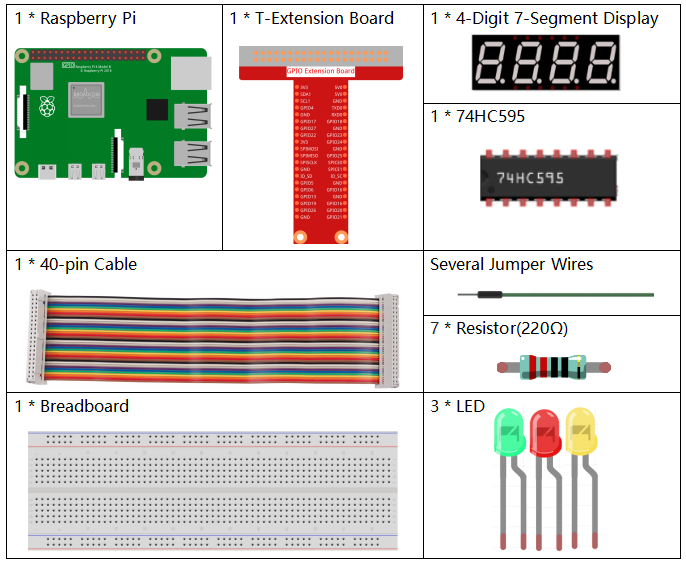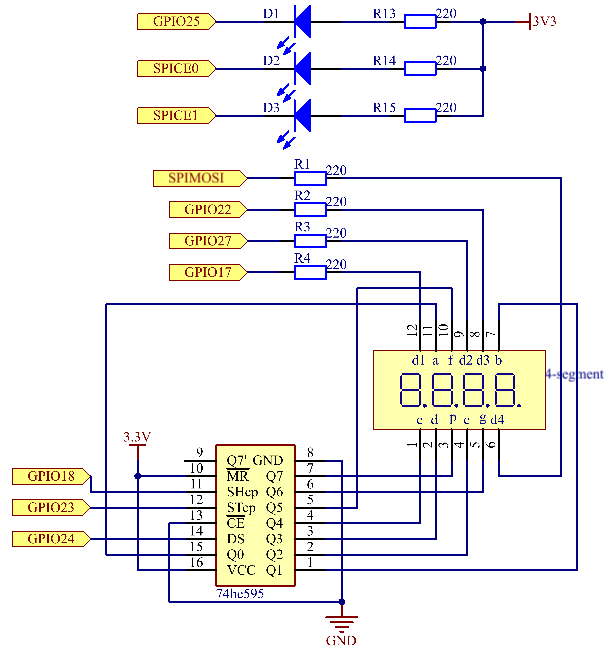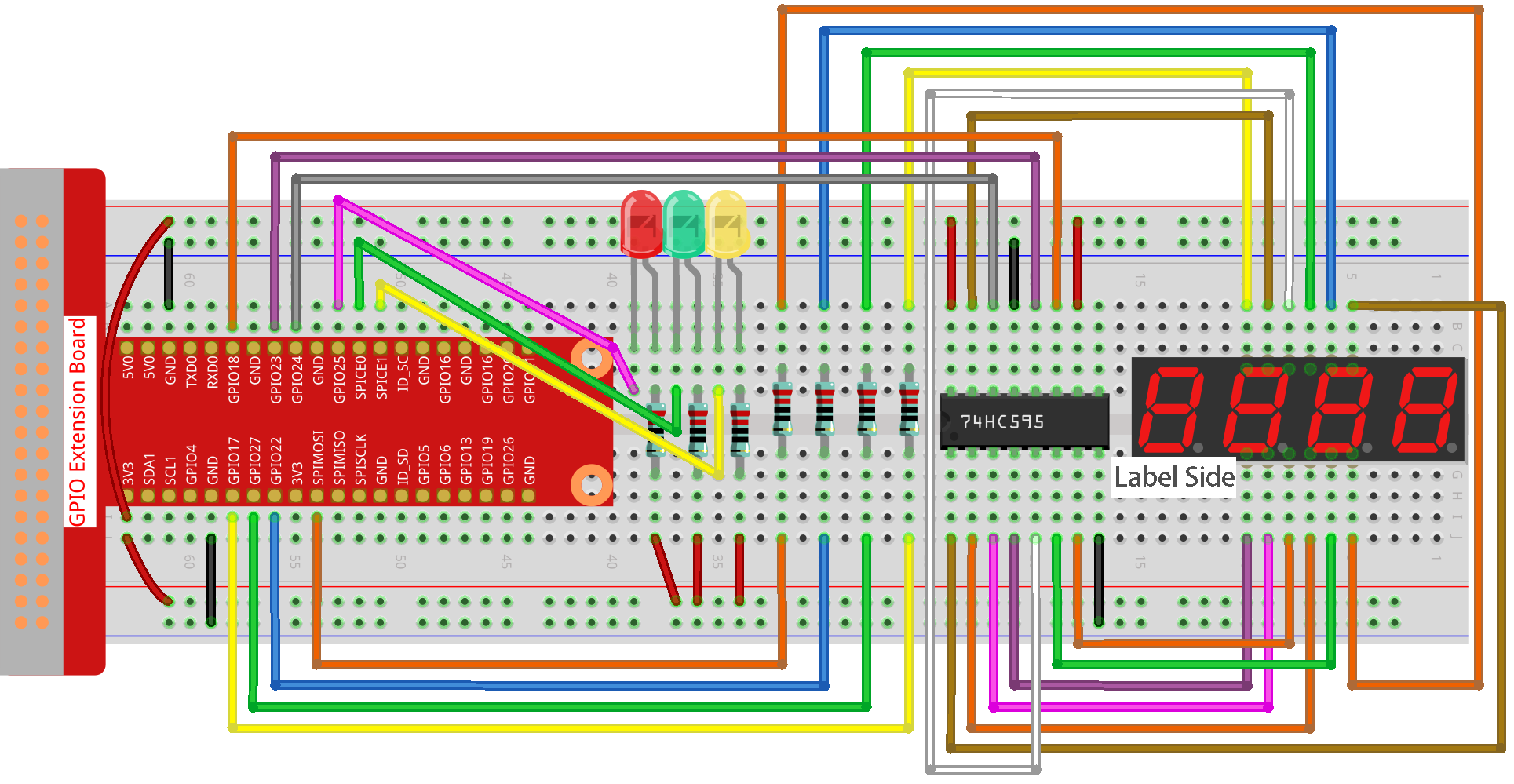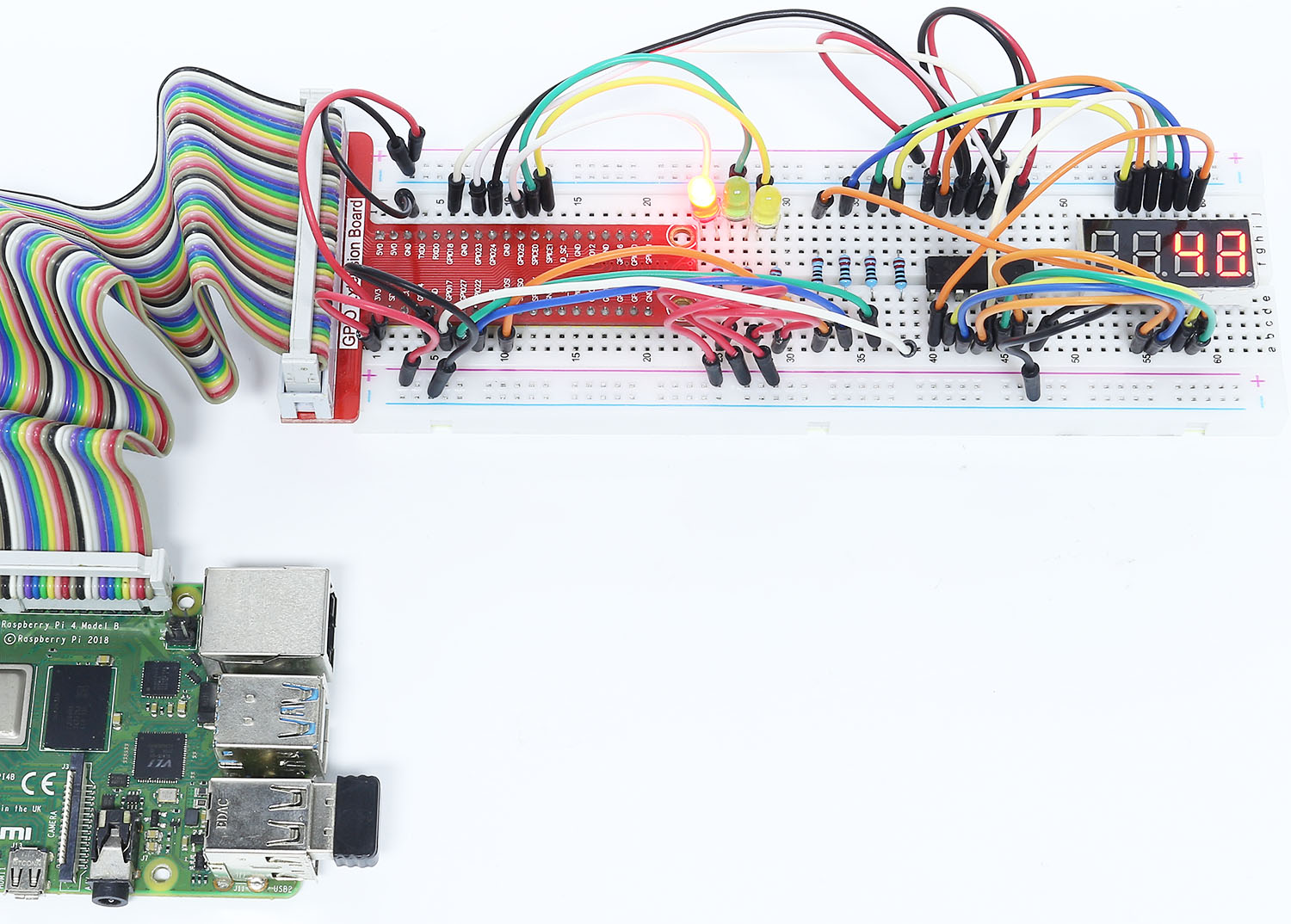# 4.1.12 Traffic Light¶

## Introduction¶

In this project, we will use LED lights of three colors to realize the change of traffic lights and a four-digit 7-segment display will be used to display the timing of each traffic state.

## Required Components¶

In this project, we need the following components.Name

ITEMS IN THIS KIT

Raphael Kit

337

Raphael Kit

COMPONENT INTRODUCTION

GPIO Extension Board

Jumper Wires

Resistor

LED

4-Digit 7-Segment Display

-

74HC595

## Schematic Diagram¶

 T-Board Name physical wiringPi BCM GPIO17 Pin 11 0 17 GPIO27 Pin 13 2 27 GPIO22 Pin 15 3 22 SPIMOSI Pin 19 12 10 GPIO18 Pin 12 1 18 GPIO23 Pin 16 4 23 GPIO24 Pin 18 5 24 GPIO25 Pin 22 6 25 SPICE0 Pin 24 10 8 SPICE1 Pin 26 11 7## Experimental Procedures¶

Step 1: Build the circuit.Step 2: Change directory.

```cd ~/raphael-kit/python/
```

Step 3: Run.

```sudo python3 4.1.12_TrafficLight.py
```

As the code runs, LEDs will simulate the color changing of traffic lights. Firstly, the red LED lights up for 60s, then the green LED lights up for 30s; next, the yellow LED lights up for 5s. After that, the red LED lights up for 60s once again. In this way, this series of actions will be executed repeatedly. Meanwhile, the 4-digit 7-segment display displays the countdown time continuously.

## Code¶

Note

You can Modify/Reset/Copy/Run/Stop the code below. But before that, you need to go to source code path like `raphael-kit/python`. After modifying the code, you can run it directly to see the effect.

```#!/usr/bin/env python3

import RPi.GPIO as GPIO
import time

#define the pins connect to 74HC595
SDI   = 24      #serial data input(DS)
RCLK  = 23     #memory clock input(STCP)
SRCLK = 18      #shift register clock input(SHCP)
number = (0xc0,0xf9,0xa4,0xb0,0x99,0x92,0x82,0xf8,0x80,0x90)

placePin = (10,22,27,17)
ledPin =(25,8,7)

greenLight = 30
yellowLight = 5
redLight = 60
lightColor=("Red","Green","Yellow")

colorState=0
counter = 60
timer1 = 0

def setup():
GPIO.setmode(GPIO.BCM)
GPIO.setup(SDI, GPIO.OUT)
GPIO.setup(RCLK, GPIO.OUT)
GPIO.setup(SRCLK, GPIO.OUT)
for pin in placePin:
GPIO.setup(pin,GPIO.OUT)
for pin in ledPin:
GPIO.setup(pin,GPIO.OUT)
global timer1
timer1.start()

def clearDisplay():
for i in range(8):
GPIO.output(SDI, 1)
GPIO.output(SRCLK, GPIO.HIGH)
GPIO.output(SRCLK, GPIO.LOW)
GPIO.output(RCLK, GPIO.HIGH)
GPIO.output(RCLK, GPIO.LOW)

def hc595_shift(data):
for i in range(8):
GPIO.output(SDI, 0x80 & (data << i))
GPIO.output(SRCLK, GPIO.HIGH)
GPIO.output(SRCLK, GPIO.LOW)
GPIO.output(RCLK, GPIO.HIGH)
GPIO.output(RCLK, GPIO.LOW)

def pickDigit(digit):
for i in placePin:
GPIO.output(i,GPIO.LOW)
GPIO.output(placePin[digit], GPIO.HIGH)

def timer():        #timer function
global counter
global colorState
global timer1
timer1.start()
counter-=1
if (counter is 0):
if(colorState is 0):
counter= greenLight
if(colorState is 1):
counter=yellowLight
if (colorState is 2):
counter=redLight
colorState=(colorState+1)%3
print ("counter : %d    color: %s "%(counter,lightColor[colorState]))

def lightup():
global colorState
for i in range(0,3):
GPIO.output(ledPin[i], GPIO.HIGH)
GPIO.output(ledPin[colorState], GPIO.LOW)

def display():
global counter

a = counter % 10000//1000 + counter % 1000//100
b = counter % 10000//1000 + counter % 1000//100 + counter % 100//10
c = counter % 10000//1000 + counter % 1000//100 + counter % 100//10 + counter % 10

if (counter % 10000//1000 == 0):
clearDisplay()
else:
clearDisplay()
pickDigit(3)
hc595_shift(number[counter % 10000//1000])

if (a == 0):
clearDisplay()
else:
clearDisplay()
pickDigit(2)
hc595_shift(number[counter % 1000//100])

if (b == 0):
clearDisplay()
else:
clearDisplay()
pickDigit(1)
hc595_shift(number[counter % 100//10])

if(c == 0):
clearDisplay()
else:
clearDisplay()
pickDigit(0)
hc595_shift(number[counter % 10])

def loop():
while True:
display()
lightup()

def destroy():   # When "Ctrl+C" is pressed, the function is executed.
global timer1
GPIO.cleanup()
timer1.cancel()      #cancel the timer

if __name__ == '__main__': # Program starting from here
setup()
try:
loop()
except KeyboardInterrupt:
destroy()
```

## Code Explanation¶

```SDI   = 24      #serial data input(DS)
RCLK  = 23     #memory clock input(STCP)
SRCLK = 18      #shift register clock input(SHCP)
number = (0xc0,0xf9,0xa4,0xb0,0x99,0x92,0x82,0xf8,0x80,0x90)
placePin = (10,22,27,17)

def clearDisplay():
def hc595_shift(data):
def pickDigit(digit):
def display():
```

These codes are used to realize the function of number display of 4-Digit 7-Segment. Refer to chapter 1.1.5 of the document for more details. Here, we use the codes to display countdown of traffic light time.

```ledPin =(25,8,7)
colorState=0

def lightup():
global colorState
for i in range(0,3):
GPIO.output(ledPin[i], GPIO.HIGH)
GPIO.output(ledPin[colorState], GPIO.LOW)
```

The codes are used to switch the LED on and off.

```greenLight = 30
yellowLight = 5
redLight = 60
lightColor=("Red","Green","Yellow")

colorState=0
counter = 60
timer1 = 0

def timer():        #timer function
global counter
global colorState
global timer1
timer1.start()
counter-=1
if (counter is 0):
if(colorState is 0):
counter= greenLight
if(colorState is 1):
counter=yellowLight
if (colorState is 2):
counter=redLight
colorState=(colorState+1)%3
print ("counter : %d    color: %s "%(counter,lightColor[colorState]))
```

The codes are used to switch the timer on and off. Refer to chapter 1.1.5 for more details. Here, when the timer returns to zero, colorState will be switched so as to switch LED, and the timer will be assigned to a new value.

```def setup():
# ...
global timer1
timer1.start()

def loop():
while True:
display()
lightup()

def destroy():   # When "Ctrl+C" is pressed, the function is executed.
global timer1
GPIO.cleanup()
timer1.cancel()      #cancel the timer

if __name__ == '__main__': # Program starting from here
setup()
try:
loop()
except KeyboardInterrupt:
destroy()
```

In `setup()` function, start the timer. In `loop()` function, a while True is used: call the relative functions of 4-Digit 7-Segment and LED circularly.

## Phenomenon Picture¶